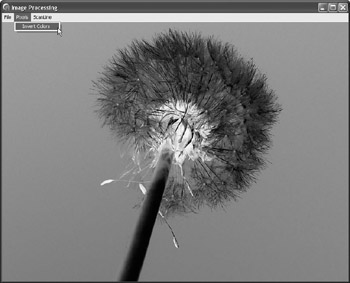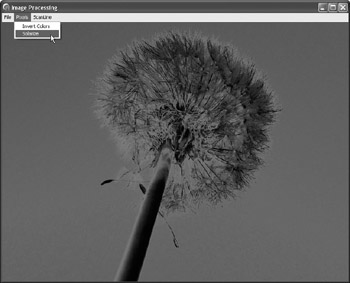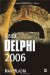The Canvas.Pixels Property

The Pixels property of the TCanvas class provides the easiest way to access pixels on a surface. It enables you to read and write the color value of a pixel at the specified location:

property Pixels[X, Y: Integer]: TColor;

Inverting Colors

To see how to use the Pixels property and how to write a simple effect, let's first create a duplicate of the InvertRect API function, which we used in Chapter 17 to enable the user to invert colors in an image.

Here are three ways to invert the pixel's color:

NewPixel = not OldPixel NewPixel = OldPixel xor 1 NewPixel = 1 -  OldPixel

To implement any of the above formulas, we must first extract the pixel's red, green, and blue values and then apply the formula to each value separately. Since the "1" in the formula represents the "maximum value," it becomes 255 in the implementation because 255 is the maximum value of a color component.

The following listing shows how to implement the NewPixel = 1 – OldPixel formula.

Listing 28-2: Inverting colors using the Pixels propertyprocedure TMainForm.PixelsInvertColorsClick(Sender: TObject); var   x: Integer;   y: Integer;   oldColor: TColor;   newRed, newGreen, newBlue: Integer; begin   for y := 0 to Pred(FImage.Height) do   begin     for x := 0 to Pred(FImage.Width) do     begin       { extract original color at x,y }       oldColor := FImage.Canvas.Pixels[x, y];       { invert the color }       newRed := 255 - GetRValue(oldColor);       newGreen := 255 - GetGValue(oldColor);       newBlue := 255 - GetBValue(oldColor);       { assign the inverted color to x,y }       FImage.Canvas.Pixels[x, y] := RGB(newRed, newGreen, newBlue);     end;         // for x   end;          // for y   Invalidate; { Invalidate the form to display the image. } end;The result of this code is displayed in Figure 28-2.Figure 28-2: The result of the Invert Colors effect

The Solarize Effect

The Solarize effect is probably the closest relative of the Invert Colors effect. To solarize an image, you have to invert a pixel's component if its value is larger than half the maximum value (127). If the value of a pixel's component is less than 127, you have to use the original value.

Here's the pseudocode of the Solarize effect:

if OldPixel > 1/2 then   NewPixel = 1 -  OldPixel else   NewPixel = OldPixel

The following listing shows how to solarize an image.

Listing 28-3: The Solarize effectprocedure TMainForm.PixelsSolarizeClick(Sender: TObject); var   x: Integer;   y: Integer;   oldColor: TColor;   newRed, newGreen, newBlue: Integer; begin   for y := 0 to Pred(FImage.Height) do   begin     for x := 0 to Pred(FImage.Width) do     begin       { extract original color at x,y }       oldColor := FImage.Canvas.Pixels[x, y];       { invert the color }       newRed := GetRValue(oldColor);       if newRed > 127 then newRed := 255 - newRed;       newGreen := GetGValue(oldColor);       if newGreen > 127 then newGreen := 255 - newGreen;       newBlue := GetBValue(oldColor);       if newBlue > 127 then newBlue := 255 - newBlue;       { assign the inverted color to x,y }       FImage.Canvas.Pixels[x, y] := RGB(newRed, newGreen, newBlue);     end;         // for x   end;          // for y   Invalidate; { Invalidate the form to display the image. } end;You can see the solarized image in the following figure.Figure 28-3: The Solarize effect

Now that you've seen and learned how to write simple effects, it's time to say goodbye to the Pixels property because it is, as you've undoubtedly noticed, extremely slow and totally inappropriate for this job.Inside Delphi 2006 (Wordware Delphi Developers Library)
ISBN: 1598220039
EAN: 2147483647
Year: 2004
Pages: 212